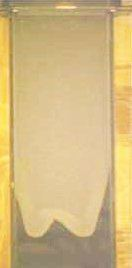# Lubrication theory

Updated on
Covid-19In fluid dynamics, lubrication theory describes the flow of fluids (liquids or gases) in a geometry in which one dimension is significantly smaller than the others. An example is the flow above air hockey tables, where the thickness of the air layer beneath the puck is much smaller than the dimensions of the puck itself.

## Contents

Internal flows are those where the fluid is fully bounded. Internal flow lubrication theory has many industrial applications because of its role in the design of fluid bearings. Here a key goal of lubrication theory is to determine the pressure distribution in the fluid volume, and hence the forces on the bearing components. The working fluid in this case is often termed a lubricant.

Free film lubrication theory is concerned with the case in which one of the surfaces containing the fluid is a free surface. In that case the position of the free surface is itself unknown, and one goal of lubrication theory is then to determine this. Surface tension may then be significant, or even dominant. Issues of wetting and dewetting then arise. For very thin films (thickness less than one micrometre), additional intermolecular forces, such as Van der Waals forces or disjoining forces, may become significant.

## Theoretical basis

Mathematically, lubrication theory can be seen as exploiting the disparity between two length scales. The first is the characteristic film thickness, H , and the second is a characteristic substrate length scale L . The key requirement for lubrication theory is that the ratio ε = H / L is small, that is, ϵ 1 . The Navier–Stokes equations (or Stokes equations, when fluid inertia may be neglected) are expanded in this small parameter, and the leading-order equations are then

p z = 0 p x = μ 2 u z 2

where x and z are coordinates in the direction of the substrate and perpendicular to it respectively. Here p is the fluid pressure, and u is the fluid velocity component parallel to the substrate; μ is the fluid viscosity. The equations show, for example, that pressure variations across the gap are small, and that those along the gap are proportional to the fluid viscosity. A more general formulation of the lubrication approximation would include a third dimension, and the resulting differential equation is known as the Reynolds equation.

Further details can be found in the literature or in the textbooks given in the bibliography.

## Applications

An important application area is lubrication of machinery components such as fluid bearings and mechanical seals. Coating is another major application area including the preparation of thin films, printing, painting and adhesives.

Biological applications have included studies of red blood cells in narrow capillaries and of liquid flow in the lung and eye.

Lubrication theory Wikipedia

Topics